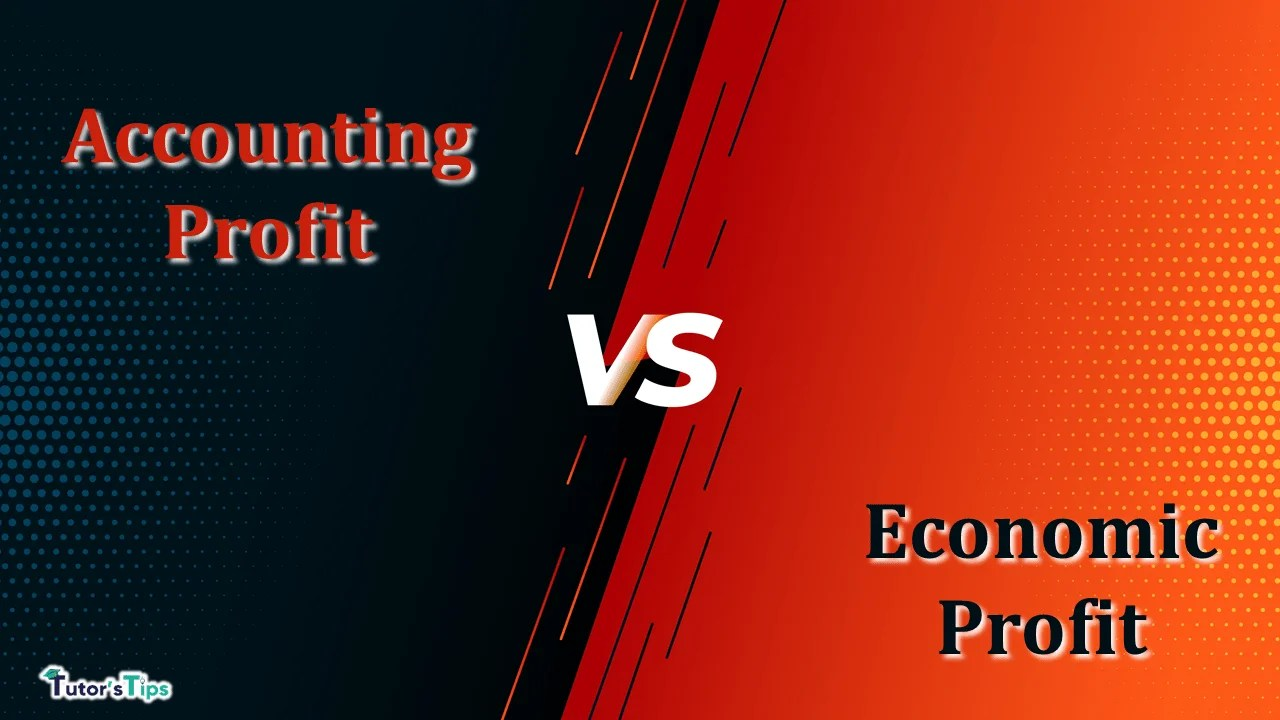# Difference between Accounting and Economic ProfitThe difference between Accounting and Economic profit is that Accounting profit refers to the net income of the business firm in a given time period. On the other hand, Economic profit refers to the remaining surplus left after deducting all costs from total revenue.

To know about the differences we have to know about the meaning of both the terms which are shown below:

## 1. Meaning of Accounting Profit:

It refers to the difference between total revenue and total costs. But, here total costs consist of only explicit costs. It can be written as:

Accounting Profit = TR- TC (includes only explicit costs)

Thus, the accountants consider only accounting profit while estimating the income of a business.

## 2. Meaning of Economic Profit:

It refers to the difference between total revenue and total costs where Total cost includes both explicit as well as implicit costs. It can be written as:

Economic Profit = TR -TC including implicit and explicit costs

In economics, we focus only on economic profits as it shows the real profitability of any business enterprise. Therefore, economic profits are considered by economists.

### The Chart of difference between Accounting and Economic Profit

 Points of differences Accounting Profit Economic Profit Meaning It refers to the net income earned by a business firm during an accounting period. It refers to the surplus obtained by deducting total costs from the total revenue. Calculation It can be calculated as the difference between Total Revenue and Total Cost where Total Cost includes the only explicit cost. It can be calculated as the difference between Total Revenue and Total Cost where TC includes both explicit as well as implicit cost. Highlights It highlights the profitability of the business firm. It highlights the efficiency of the business firm in the allocation of resources. Relevance Accounting profit is practical from the viewpoint of the financial perspective. Economic profit doesn’t provide a precise picture. Considered By It is considered by the accountants. It is considered by economists. Formula Accounting Profit = Total Revenue – Explicit costs Economic Profit = Total Revenue -(Explicit + Implicit Costs) Consideration It doesn’t consider Opportunity Costs. It considers Opportunity Costs. Inter – Relation Accounting profit is normally more than economic profit. Economic profit is normally less than accounting profit. Can also be referred to It can also be referred to  as the revenue obtaining post-meeting all economic costs It can also be referred to as the profit when total costs exceed opportunity costs. Relevance in the Long run It is basically a short-run phenomenon as it tells the financial health of the business. It is used for long-term strategies in business. Reliability It is the real profit of the firm. It is the abnormal profit of the business firm.

## Conclusion:

Thus, the accounting profit represents the profitability of the firm. As the accountant considers it while estimating production costs and other costs. On the other hand, Economic profit represents the efficiency of a business firm.